# Convergence Diagnostics

In :
%matplotlib inline
import numpy as np
from scipy.stats import norm,uniform
import matplotlib.pyplot as plt
import seaborn as sbn
import pandas as pd
import warnings
warnings.filterwarnings('ignore')

np.random.seed(282629734)

sbn.set_style('white')
sbn.set_context('talk')


## The Convergence Problem¶

From the previous notebook, we know that using MH leads to a Markov Chain that we can use for inference. This is predicated on our chain converging to our stationary distribution (the posterior). Knowing when a chain has converged is a numerical issue and there are some important diagnostic tools we'll be using for assessing convergence.

For having some data to play with, let's resurrect our simple example yet again:

In :
data = norm(10,3).rvs(10)
sigma = 3          # Note this is the std of x
mu_prior = 8
sigma_prior = 1.5  # Note this is our prior on the std of mu

plt.hist(data,bins=20)
plt.show()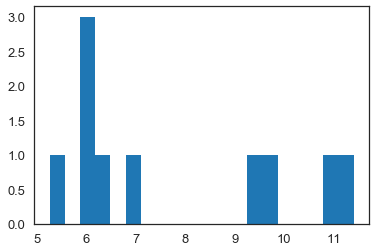In :
# a fairly basic mh mcmc random walk sampler:
def sampler(data, samples=4, mu_init=9, sigma= 1, proposal_width=3,
mu_prior_mu=10, mu_prior_sd=1):
mu_current = mu_init
posterior = [mu_current]
for i in range(samples):
# suggest new position
mu_proposal = norm(mu_current, proposal_width).rvs()

# Compute likelihood by multiplying probabilities of each data point
likelihood_current = norm(mu_current, sigma).pdf(data).prod()
likelihood_proposal = norm(mu_proposal, sigma).pdf(data).prod()

# Compute prior probability of current and proposed mu
prior_current = norm(mu_prior_mu, mu_prior_sd).pdf(mu_current)
prior_proposal = norm(mu_prior_mu, mu_prior_sd).pdf(mu_proposal)

p_current = likelihood_current * prior_current
p_proposal = likelihood_proposal * prior_proposal

# Accept proposal?
c = np.min((1,p_proposal / p_current))

accept = np.random.rand() <= c

if accept:
# Update position
mu_current = mu_proposal

posterior.append(mu_current)

return np.array(posterior)

In :
chain = sampler(data,samples=5000,mu_init=9,sigma=sigma,proposal_width=2,
mu_prior_mu=mu_prior,mu_prior_sd = sigma_prior)

In :
plt.figure(figsize=(15,6))
plt.subplot(121)
plt.plot(np.arange(chain.shape),chain)
plt.title('Trace Plot for $\\mu$')

plt.subplot(122)
plt.hist(chain,orientation='horizontal',bins=30)
plt.title('Histogram for $\\mu$')

plt.tight_layout()
plt.show()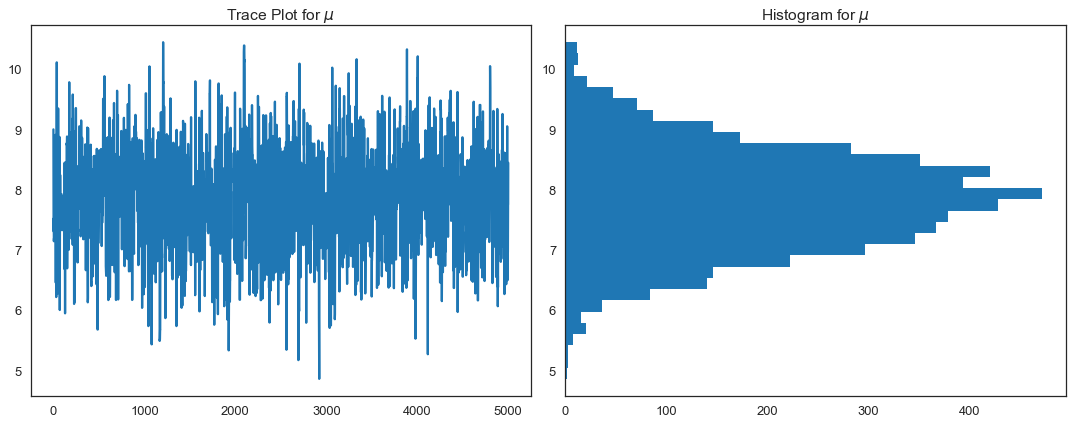## How do we know this chain has converged to the posterior?¶

### Standard Error of the Mean¶

This investigates the question how does the mean of $\theta$ deviate in our chain, and is capturing the simulation error of the mean rather than underlying uncertainty of our parameter $\theta$:

$$SE^{\bar{\theta}} = \frac{\text{Posterior Standard Deviation}}{\sqrt{L}}$$

where $L$ is the chain length (the number of iterations in your chain). Note that the package we'll be using calls this MC Error.

For our problem, this is:

In :
print("Standard Error of the Mean: ", chain.std()/np.sqrt(chain.shape))

Standard Error of the Mean:  0.011656241240510384


This is saying that very little of our posterior variation in $\theta$ is due to sampling error (that is good). We can visualize this by examining the moving average of our chain as we move through the 5000 iterations:

In :
# pandas makes this easy:
df_chain = pd.DataFrame(chain,columns=['theta'])
df_chain['ma_theta'] = df_chain.theta.rolling(window=100,center=False).mean()
#df_chain['ma_theta'] = pd.stats.moments.rolling_mean(df_chain.theta,100)
df_chain['iteration'] = df_chain.index.values
ax = df_chain.plot(x=['iteration'],y=['theta','ma_theta'],figsize=(15,7))
ax.lines[-1].set_linewidth(4)
ax.lines[-1].set_color('k')
ax.lines.set_label("$\\theta$")
ax.lines[-1].set_label("Moving Average of $\\theta$")
plt.legend()
plt.show()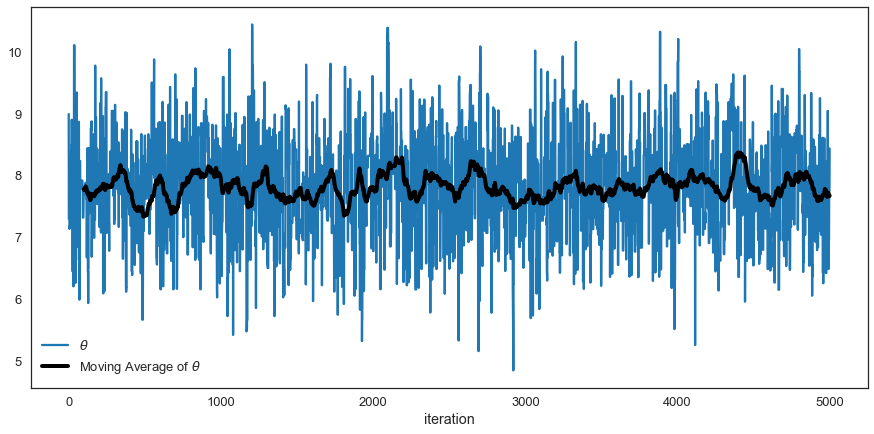This is a good sign that our chain is stable, since both the individual samples of $\theta$ in our chain and the mean of the samples dance around a stable value of $\theta$. The calculation above makes this more concrete. There are time series versions of this calculation that accounts for the fact that the chain is not iid.

### Autocorrelation Plots¶

In :
import pymc3 as pm3

lags=np.arange(1,100)

fig, ax = plt.subplots()
ax.plot(lags, [pm3.autocorr(chain, l) for l in lags])
_ = ax.set(xlabel='lag', ylabel='autocorrelation', ylim=(-.1, 1))
plt.title('Autocorrelation Plot')
plt.show()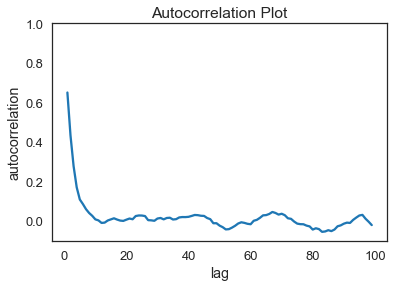### Acceptance Rate for the MH Algorithm¶

Recall that we want the acceptance rate to be in the range .2 to .4. For our problem this paper suggests an acceptance rate of .234 for random walk MH.

Since the number of new members in the chain represent the number of acceptances, count changes in chain values and divide by total chain length to calculate acceptance rate:

In :
changes = np.sum((chain[1:]!=chain[:-1]))
print("Acceptance Rate is: ", changes/(chain.shape-1))

Acceptance Rate is:  0.4232


The acceptance rate is helpful in describing convergence because it indicates a good level of "mixing" over the parameter space. Here the acceptance rate is too low, so we should decrease proposal width and re-run our MH MCMC sampler.

Note: modern software (like pymc) can auto-tune $\omega$ to achieve a desired acceptance rate.

### Geweke Diagnostic¶

We can explicitly think of this test as a test for the Ergodicity (stationarity) of your chain.

Take the first 10 and last 50% of your chain and do a z test comparing means (correcting for autocorrelation). Software packages, take this a step further: The geweke function in pymc3 by default chooses the first 10% of your chain, and the final 50%; divides the final 50% of the chain into 20 segments and performs a z-test for each segment. You want to fail to reject the null, since the hypothesis is:

$$H_0: \theta_{10\%} = \theta^s_{50\%} \\ H_1: \theta_{10\%} \ne \theta^s_{50\%}$$

for each segment $s$. If our means are the same (we fail to reject the null), then we have strong evidence of chain convergence.

In :
gw_plot = pm3.geweke(chain)
plt.scatter(gw_plot[:,0],gw_plot[:,1])
plt.axhline(-1.98, c='r')
plt.axhline(1.98, c='r')
plt.ylim(-2.5,2.5)
plt.title('Geweke Plot Comparing first 10% and Slices of the Last 50% of Chain')
plt.xlim(-10,2510)
plt.show()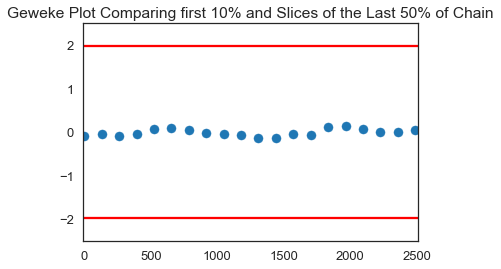Even without dropping any burn-in observations, we have convergence. We only start seeing issues when we restrict ourselves to the first 5 values in the chain. Suggests we should drop the first few dozen observations for burn-in.

In :
gw_plot = pm3.geweke(chain,.001,.5,20)
plt.scatter(gw_plot[:,0],gw_plot[:,1])
plt.axhline(-1.98, c='r')
plt.axhline(1.98, c='r')
plt.ylim(-2.5,2.5)
plt.xlim(-10,2510)
plt.title('Geweke Plot Comparing first .1% and Slices of the Last 50% of Chain')
plt.show()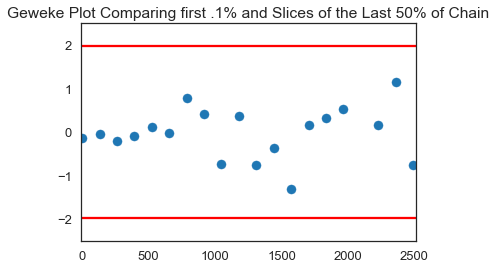### Gelman Rubin Diagnostic¶

If our MH MCMC Chain reaches a stationary distribution, and we repeat the excercise multiple times, then we can examine if the posterior for each chain converges to the same place in the distribution of the parameter space.

Steps:

1. Run $M>1$ Chains of length $2 \times N$.
2. Discard the first $N$ draws of each chain, leaving $N$ iterations in the chain.
3. Calculate the within and between chain variance.

• Within chain variance: $$W = \frac{1}{M}\sum_{j=1}^M s_j^2$$ where $s_j^2$ is the variance of each chain (after throwing out the first $N$ draws).
• Between chain variance: $$B = \frac{N}{M-1} \sum_{j=1}^M (\bar{\theta_j} - \bar{\bar{\theta}})^2$$

where $\bar{\bar{\theta}}$ is the mean of each of the M means.

4. Calculate the estimated variance of $\theta$ as the weighted sum of between and within chain variance. $$\hat{var}(\theta) = \left ( 1 - \frac{1}{N}\right ) W + \frac{1}{N}B$$
5. Calculate the potential scale reduction factor. $$\hat{R} = \sqrt{\frac{\hat{var}(\theta)}{W}}$$

We want this number to be close to 1. Why? This would indicate that the between chain variance is small. This makes sense, if between chain variance is small, that means both chains are mixing around the stationary distribution. Gelmen and Rubin show that when $\hat{R}$ is greater than 1.1 or 1.2, we need longer burn-in.

Let's run 2 chains:

In :
chain1 = sampler(data,samples=5000,mu_init=9,sigma=sigma,proposal_width=2,
mu_prior_mu=mu_prior,mu_prior_sd = sigma_prior)
chain2 = sampler(data,samples=5000,mu_init=9,sigma=sigma,proposal_width=2,
mu_prior_mu=mu_prior,mu_prior_sd = sigma_prior)

In :
plt.figure(figsize=(15,5))
plt.plot(np.arange(5001),chain1)
plt.plot(np.arange(5001),chain2,alpha=.7)

Out:
[<matplotlib.lines.Line2D at 0x7fd2f6e9add8>]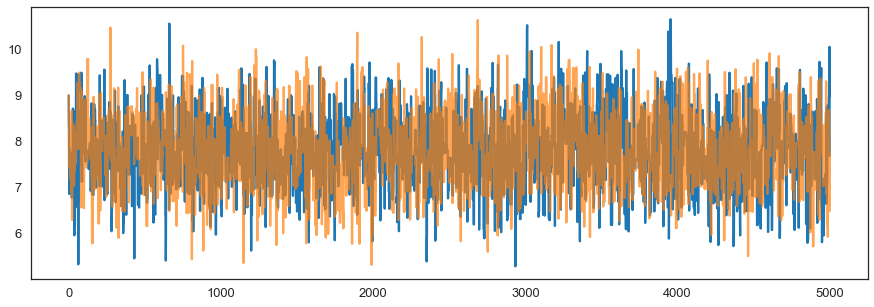In :
# note, I can't use pm3.gelmen_rubin() because my chains are not pymc trace objects :-(
burn_in = 10
length = 10

n = chain1[burn_in:burn_in+length].shape

W = (chain1[burn_in:burn_in+length].std()**2 + chain2[burn_in:burn_in+length].std()**2)/2
mean1 = chain1[burn_in:burn_in+length].mean()
mean2 = chain2[burn_in:burn_in+length].mean()
mean = (mean1 + mean2)/2
B = n * ((mean1 - mean)**2 + (mean2 - mean)**2)
var_theta = (1 - 1/n) * W + 1/n*B
print("Gelmen-Rubin Diagnostic: ", np.sqrt(var_theta/W))

Gelmen-Rubin Diagnostic:  1.161636365352385


It should be evident that Gelmen-Rubin has two factors at play: burn-in and chain length. Really this criteria can be used ex-post to justify decisions on burn-in and chain length.

## Univariate Approaches¶

The diagnostics we have discussed are all univariate (they work perfectly when there is only 1 parameter to estimate). Other diagnostics have been derived for the multivariate case, but these are useful only when using Gibbs Samplers or other specialized versions of Metropolis-Hastings.

So most people examine univariate diagnostics for each variable, examine autocorrelation plots, acceptance rates and try to argue chain convergence based on that- unless they are using Gibbs or other specialized samplers.